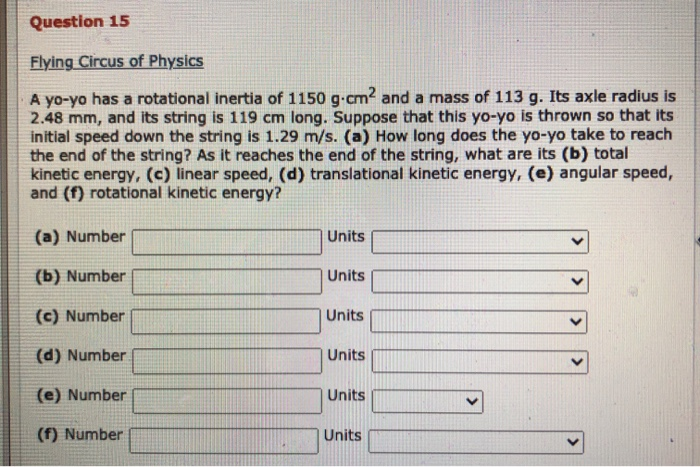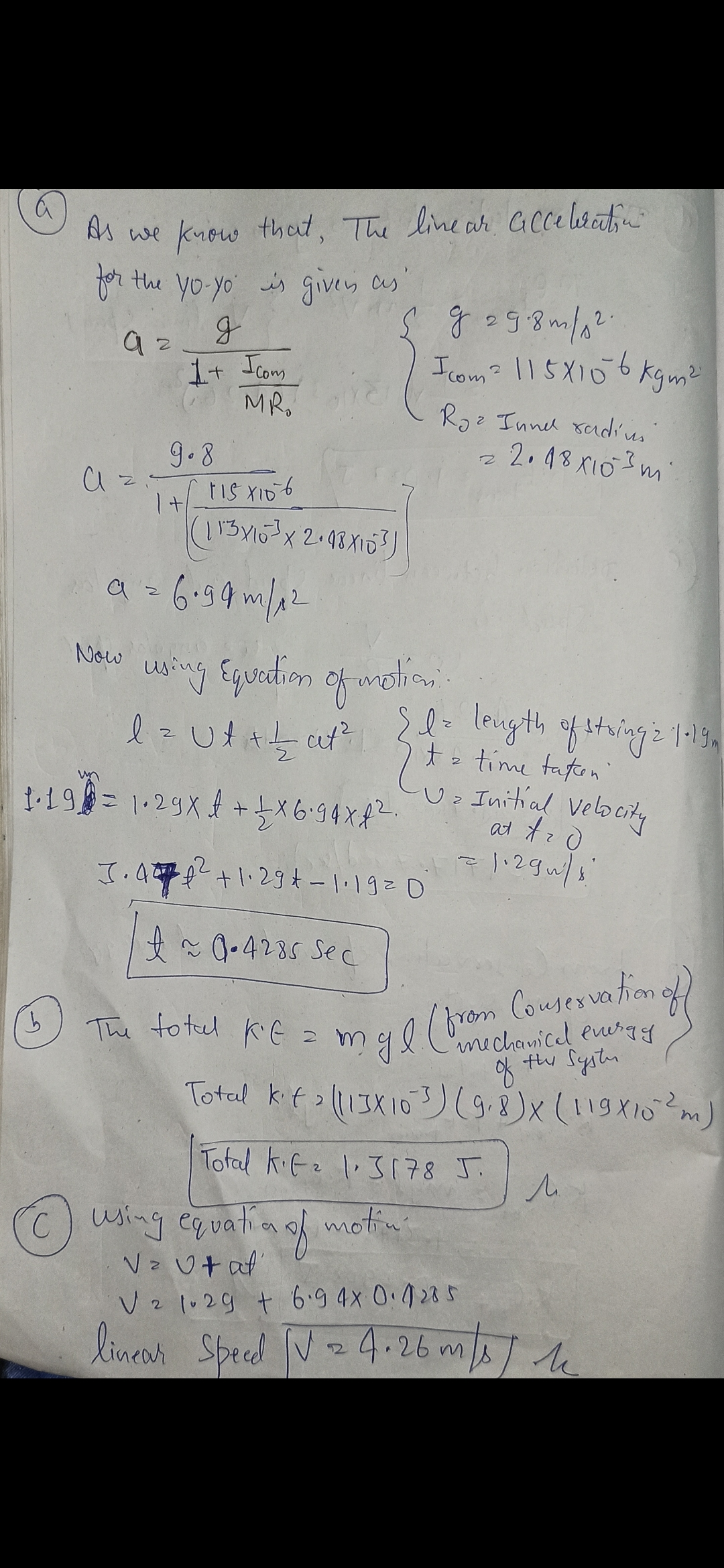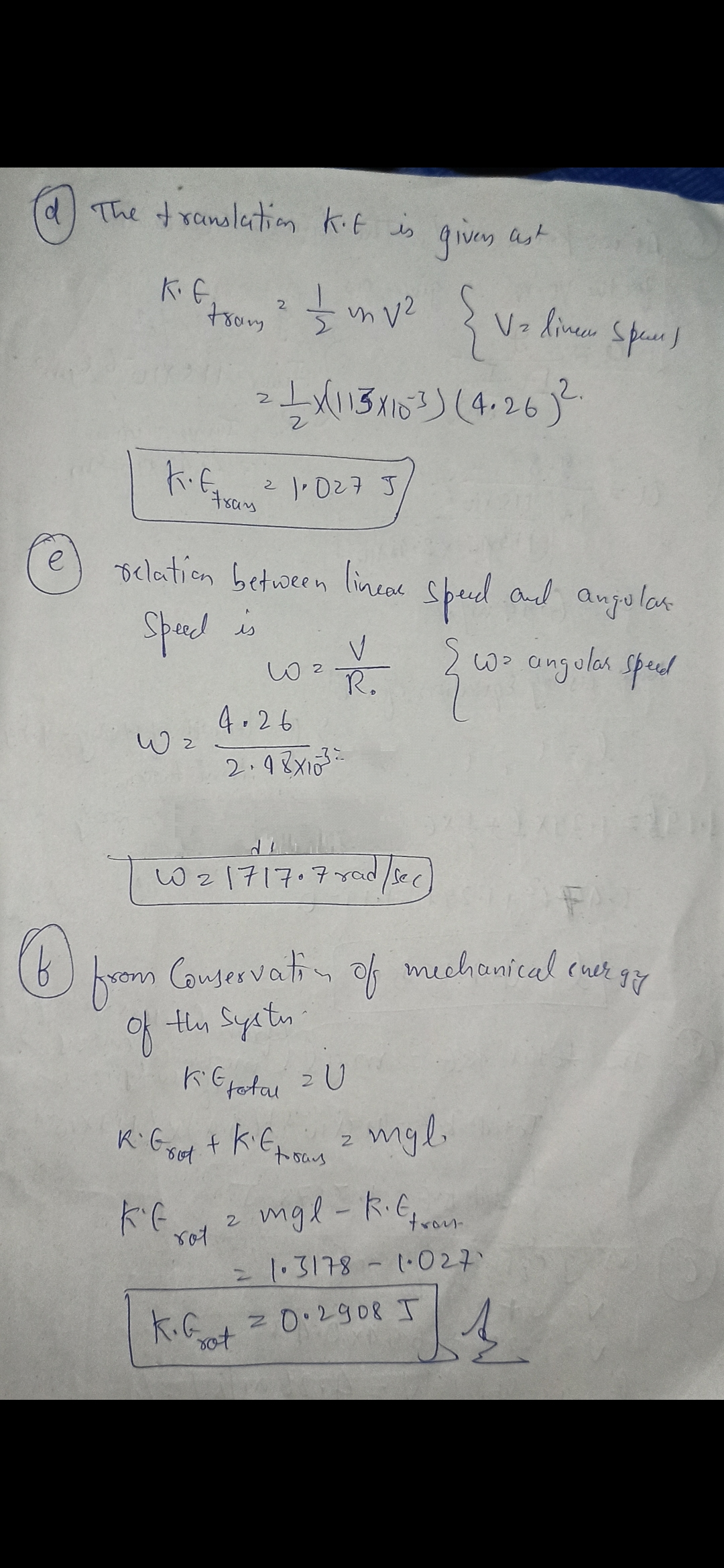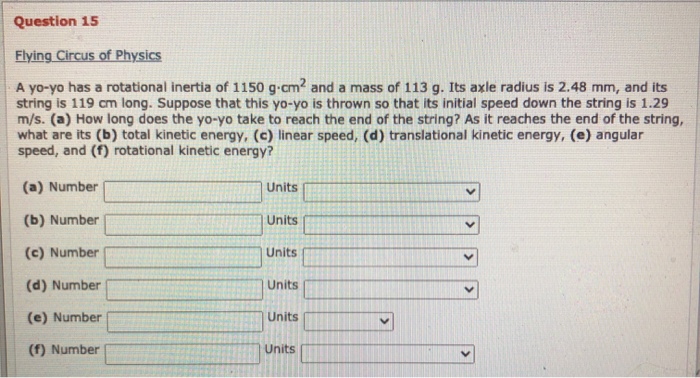# Question 15 Flying Circus of Physics A yo-yo has a rotational inertia of 1150 g.cm2 and...Question 15 Flying Circus of Physics A yo-yo has a rotational inertia of 1150 g.cm2 and a mass of 113 g. Its axle radius is 2.48 mm, and its string is 119 cm long. Suppose that this yo-yo is thrown so that its initial speed down the string is 1.29 m/s. (a) How long does the yo-yo take to reach the end of the string? As it reaches the end of the string, what are its (b) total kinetic energy, (c) linear speed, (d) translational kinetic energy, (e) angular speed, and (f) rotational kinetic energy? (a) Number Units (b) Number Units (c) Number Units (d) Number Units (e) Number Units (f) Number UnitsKindly thumbs up?. Any doubt ask me. Thanks dear :)

##### Add Answer of: Question 15 Flying Circus of Physics A yo-yo has a rotational inertia of 1150 g.cm2 and...
Similar Homework Help Questions
• ### Question 15 Flying Circus of Physics A yo-yo has a rotational inertia of 1150 g.cmand a...Question 15 Flying Circus of Physics A yo-yo has a rotational inertia of 1150 g.cmand a mass of 113 g. Its axle radius is 2.48 mm, and its string is 119 cm long. Suppose that this yo-yo is thrown so that its initial speed down the string is 1.29 m/s. (a) How long does the yo-yo take to reach the end of the string? As it reaches the end of the string, what are its (b) total kinetic energy, (c)...

• ### Yo-yo help me please

A yo-yo has a rotational inertia of 900 g·cm2 and a mass of 100 g. Its axle radius is 3.3 mm and its string is 100 cm long. The yo-yo is thrown so that its its initialspeed down the string is 1.2 m/s.(a) How long does the yo-yo take to reach the end of the string?___________s(b) As it reaches the end of the string, what is its total kinetic energy?___________J(c) As it reaches the end of the string, what is...

• ### Yo yo need help with yo-yo

A yo-yo has a rotational inertia of 1000 g·cm2 and a mass of 110 g. Its axle radius is 3.1 mm and its string is 100 cm long. The yo-yo is thrown so that its itsinitial speed down the string is 1.0 m/s. As the yo-yo reaches the end of the string, what are the following values?(a) the time taken to reach the end of the string in seconds(b) its total kinetic energy Joules(c) its linear speed in meters/second(d) its...

• ### Physics

A yo-yo has a rotational inertia of 790 g·cm2 and a mass of 95 g. Its axle radius is 2.2 mm and its string is 100 cm long. The yo-yo is thrown so that its itsinitial speed down the string is 1.2 m/s.(b) As it reaches the end of the string, what is its total kinetic energy?d) As it reaches the end of the string, what is its translational kinetic energy?(f) As it reaches the end of the string, what...

• ### Physics

A yo-yo has a rotational inertia of 790 g·cm2 and a mass of 95 g. Its axle radius is 2.2 mm and its string is 100 cm long. The yo-yo is thrown so that its its initialspeed down the string is 1.2 m/s.(a) How long does the yo-yo take to reach the end of the string?(b) As it reaches the end of the string, what is its total kinetic energy?(c) As it reaches the end of the string, what is...

• ### yo-yo

Question details:A yo-yo has a rotational inertia of 757 g·cm2 anda mass of 102 g. Its axle radius is 3.08 mm, and its string is 102cm long. The yo-yo rolls from rest downto the end of the string.(a) What is the magnitude of its linearacceleration? (b) How long does it take to reachthe end of thestring? As it reaches the end of the string, whatare its (c) linear speed, (d)translational kinetic energy, (e)rotationalkinetic energy, and (f) angular speed?Thanks

• ### pls

A yo-yo has a rotational inertia of 800 g·cm2 and a mass of 125 g. Its axle radius is 2.6 mm and its string is 120 cm long. The yo-yo is thrown so that its its initialspeed down the string is 1.2 m/s. As the yo-yo reaches the end of the string, what are the following values?(a) the time taken to reach the end of the string(b) its total kinetic energy(c) its linear speed(d) its translational kinetic energy(e) its angular...

• ### physics

A yo-yo has a rotational inertia of 1190 g·cm2 and a mass of 180 g. Its axle radius is 3.9 mm, and its string is 140 cm long. The yo-yo rolls from rest down to the endof the string.(a) What is the magnitude of its linear acceleration?(b) How long does it take to reach the end of the string?(c) As it reaches the end of the string, what is its linear speed?(d) As it reaches the end of the string,...

• ### Physics Questions

A yo-yo has a rotational inertia of 1070 g·cm2and a mass of 102 g. Its axle radius is 2.91 mm, and its string is133 cm long. The yo-yo rolls from rest downto the end of thestring. (a) What is the magnitude of its linearacceleration? (b) How long does it take to reachthe end of thestring? As it reaches the end of the string, whatare its (c) linear speed, (d)translational kinetic energy, (e)rotationalkinetic energy, and (f) angular speed?

• ### Rolling, Torque, and Angular Momentum Help!!! Due Tonight!!!

A yo-yo has a rotational inertia of 960 g·cm2 and a mass of 100 g. Its axle radius is 2.3 mm and its string is 115 cm long. The yo-yo is thrown so that its its initialspeed down the string is 1.5 m/s. As the yo-yo reaches the end of the string, what are the following values?(a) the time taken to reach the end of the strings(b) its total kinetic energyJ(c) its linear speedm/s(d) its translational kinetic energyJ(e) its angular...

Free Homework App